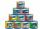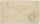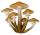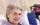Ravens

The tale of the Seven Ravens were seven brothers, each of whom was born exactly 2.5 years after the previous one. When the eldest of the brothers was 2-times older than the youngest, mother all curse. How old was seven ravens brothers when their mother cursed?

Result

k1 (youngest) =  15
k2 =  17.5
k3 =  20
k4 =  22.5
k5 =  25
k6 =  27.5
k7 (oldest) =  30

Solution:Leave us a comment of this math problem and its solution (i.e. if it is still somewhat unclear...):Be the first to comment!To solve this verbal math problem are needed these knowledge from mathematics:

Do you have a linear equation or system of equations and looking for its solution? Or do you have quadratic equation?

Next similar math problems:

1. Walnutsx walnuts were in the mission. Dano took 1/4 of nuts Michael took 1/8 from the rest and John took 34 nuts. It stayed here 29 nuts. Determine the original number of nuts.
2. Apples 2How many apples were originally on the tree, if the first day fell one third, the second day quarter of the rest and on tree remained 45 apples?
3. NormThree workers planted 3555 seedlings of tomatoes in one dey. First worked at the standard norm, the second planted 120 seedlings more and the third 135 seedlings more than the first worker. How many seedlings were standard norm?
4. CansHow many cans must be put in the bottom row if we want 182 cans arrange in 13 rows above so that each subsequent row has always been one tin less? How many cans will be in the top row?
5. SeatsSeats in the sport hall are organized so that each subsequent row has five more seats. First has 10 seats. How many seats are: a) in the eighth row b) in the eighteenth row
6. Simple equationSolve the following simple equation: 2. (4x + 3) = 2-5. (1-x)
7. CakesGrandmother baked cakes. Half of its was poppy, quarter with plum jam and 16 cheesecakes. How many cakes she baked in total?
8. Forest nurseryIn the forest nursery after winter, they found that 1/10 stems died out of them. For them, they land 193 new spruces. How many spruces are in the forest nursery?
9. AuntAnnie aunt is 35 years old, Annie is 14 years. For how many years will aunt have twice as many years as Annie?
10. MushroomsFor five days, we have collected 410 mushrooms. Interestingly every day we have collected 10 mushrooms more than the preceding day. How many mushrooms we have collected during 4th day?
11. FamilyMom is 39 years old. Karl is eight years and Paul 4 years. For many years mother would old just like two children together?
12. How oldThe student who asked how many years he answered: "After 10 years I will be twice as old than as I was four years ago. How old is student?
13. Unknown number 11That number increased by three equals three times itself?
14. Father and sonFather is 48 years old, son 23. When will be the father twice older than a son?
15. Equation 29Solve next equation: 2 ( 2x + 3 ) = 8 ( 1 - x) -5 ( x -2 )
16. FactoryIn the factory workers work in three shifts. In the first inning operates half of all employees in the second inning and a third in the third inning 200 employees. How many employees work at the factory?
17. Sequence 2Write the first 5 members of an arithmetic sequence a11=-14, d=-1